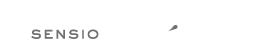Fork me on GitHub# Rule `cast_spaces`¶

A single space or none should be between cast and variable.

## Configuration¶

### `space`¶

Spacing to apply between cast and variable.

Allowed values: `'none'` and `'single'`

Default value: `'single'`

## Examples¶

### Example #1¶

Default configuration.

```--- Original
+++ New
<?php
-\$bar = ( string )  \$a;
-\$foo = (int)\$b;
+\$bar = (string) \$a;
+\$foo = (int) \$b;
```

### Example #2¶

With configuration: `['space' => 'single']`.

```--- Original
+++ New
<?php
-\$bar = ( string )  \$a;
-\$foo = (int)\$b;
+\$bar = (string) \$a;
+\$foo = (int) \$b;
```

### Example #3¶

With configuration: `['space' => 'none']`.

```--- Original
+++ New
<?php
-\$bar = ( string )  \$a;
-\$foo = (int) \$b;
+\$bar = (string)\$a;
+\$foo = (int)\$b;
```

## Rule sets¶

The rule is part of the following rule sets:

## Source class¶

PhpCsFixer\Fixer\CastNotation\CastSpacesFixer